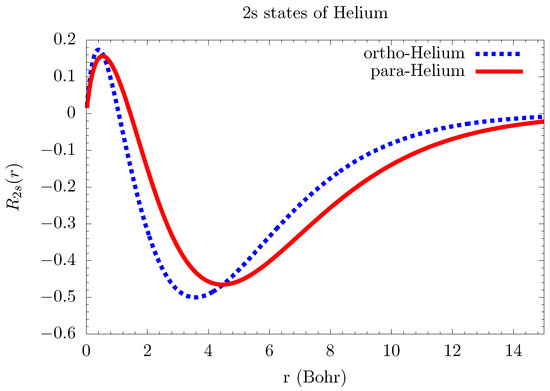Next Article in Journal / Special Issue
Revisiting the Stark Width and Shift of He II Pα

Metrics 0

## Export Article

Open AccessFeature PaperArticle

# Matrix Methods for Solving Hartree-Fock Equations in Atomic Structure Calculations and Line Broadening

1
Sandia National Laboratories, Albuquerque, NM 87123, USA
2
Los Alamos National Laboratory, Los Alamos, NM 87545, USA
3
Department of Astronomy, University of Texas at Austin, Austin, TX 78712, USA
*
Author to whom correspondence should be addressed.
Atoms 2018, 6(2), 22; https://doi.org/10.3390/atoms6020022
Received: 7 March 2018 / Revised: 14 April 2018 / Accepted: 16 April 2018 / Published: 23 April 2018
(This article belongs to the Special Issue Spectral Line Shapes in Plasmas II)
|
PDF [398 KB, uploaded 3 May 2018]
|

# Abstract

Atomic structure of N-electron atoms is often determined by solving the Hartree-Fock equations, which are a set of integro-differential equations. The integral part of the Hartree-Fock equations treats electron exchange, but the Hartree-Fock equations are not often treated as an integro-differential equation. The exchange term is often approximated as an inhomogeneous or an effective potential so that the Hartree-Fock equations become a set of ordinary differential equations (which can be solved using the usual shooting methods). Because the Hartree-Fock equations are an iterative-refinement method, the inhomogeneous term relies on the previous guess of the wavefunction. In addition, there are numerical complications associated with solving inhomogeneous differential equations. This work uses matrix methods to solve the Hartree-Fock equations as an integro-differential equation. It is well known that a derivative operator can be expressed as a matrix made of finite-difference coefficients; energy eigenvalues and eigenvectors can be obtained by using linear-algebra packages. The integral (exchange) part of the Hartree-Fock equation can be approximated as a sum and written as a matrix. The Hartree-Fock equations can be solved as a matrix that is the sum of the differential and integral matrices. We compare calculations using this method against experiment and standard atomic structure calculations. This matrix method can also be used to solve for free-electron wavefunctions, thus improving how the atoms and free electrons interact. This technique is important for spectral line broadening in two ways: it improves the atomic structure calculations, and it improves the motion of the plasma electrons that collide with the atom. View Full-Text
Keywords:
FiguresFigure 1

This is an open access article distributed under the Creative Commons Attribution License which permits unrestricted use, distribution, and reproduction in any medium, provided the original work is properly cited (CC BY 4.0).MDPI and ACS Style

Gomez, T.; Nagayama, T.; Fontes, C.; Kilcrease, D.; Hansen, S.; Montgomery, M.; Winget, D. Matrix Methods for Solving Hartree-Fock Equations in Atomic Structure Calculations and Line Broadening. Atoms 2018, 6, 22.

Note that from the first issue of 2016, MDPI journals use article numbers instead of page numbers. See further details here.

1